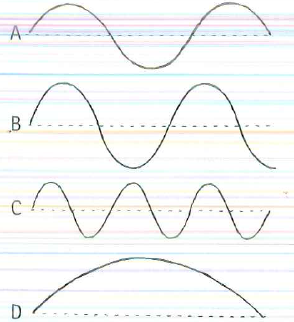# Problem: All the waves shown have the same speed in the same medium. Use a ruler and rank these waves from most to least fora. amplitude.b. wavelength.c. frequency.d. period.

###### FREE Expert Solution

We'll organize all these variables in a table.

We'll use a ruler to measure the amplitude.

We'll also use a ruler to measure the length occupied by a given number of wavelengths. The wavelength is given by:

We can also measure the wavelength directly if the waveforms a full wavelength.

87% (55 ratings)###### Problem Details

All the waves shown have the same speed in the same medium. Use a ruler and rank these waves from most to least for

a. amplitude.
b. wavelength.
c. frequency.
d. period.